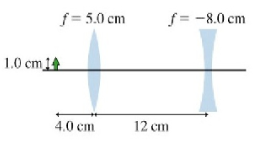# Problem: The figure shows a combination of two lensesa. Find the position of the final image of the 1.0 cm tall objectb. Find the size of the final image of the 1.0-cm-tall objectc. Find the orientation of the final image of the 1.0-cm-tall object

###### FREE Expert Solution

Lens maker equation:

$\overline{)\frac{\mathbf{1}}{{\mathbf{s}}_{\mathbf{o}}}{\mathbf{+}}\frac{\mathbf{1}}{{\mathbf{s}}_{\mathbf{i}}}{\mathbf{=}}\frac{\mathbf{1}}{\mathbf{f}}}$

Magnification:

$\overline{){\mathbf{m}}{\mathbf{=}}{\mathbf{-}}\frac{{\mathbf{s}}_{\mathbf{i}}}{{\mathbf{s}}_{\mathbf{o}}}}$

a.

From the lens maker equation:

1/s1i = 1/f1 - 1/s1o = 1/5 - 1/4 = - 1/20###### Problem Details

The figure shows a combination of two lensesa. Find the position of the final image of the 1.0 cm tall object

b. Find the size of the final image of the 1.0-cm-tall object

c. Find the orientation of the final image of the 1.0-cm-tall object